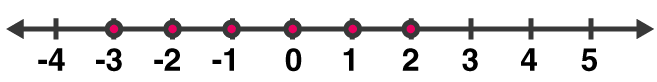# ML Aggarwal Solutions for Class 7 Maths Chapter 9 Linear Equations and Inequalities

ML Aggarwal Solutions for Class 7 Maths Chapter 9 Linear Equations and Inequalities can be utilized by the students to understand the concepts in a descriptive manner. The solutions are prepared by experts, keeping in mind the understanding abilities of students. Further, these solutions are in accordance with the current ICSE board guidelines. In order to obtain conceptual knowledge, students can download ML Aggarwal Solutions for Class 7 Maths Chapter 9 Linear Equations and Inequalities PDF, from the links given below

Chapter 9 discusses all the fundamental concepts of Linear Equations and Inequalities which are important from an exam point of view. Students are highly recommended to refer to ML Aggarwal Solutions for better performance in academics. These solutions are available online and offline, as per the students requirement.

## ML Aggarwal Solutions for Class 7 Maths Chapter 9 Linear Equations and Inequalities Download PDF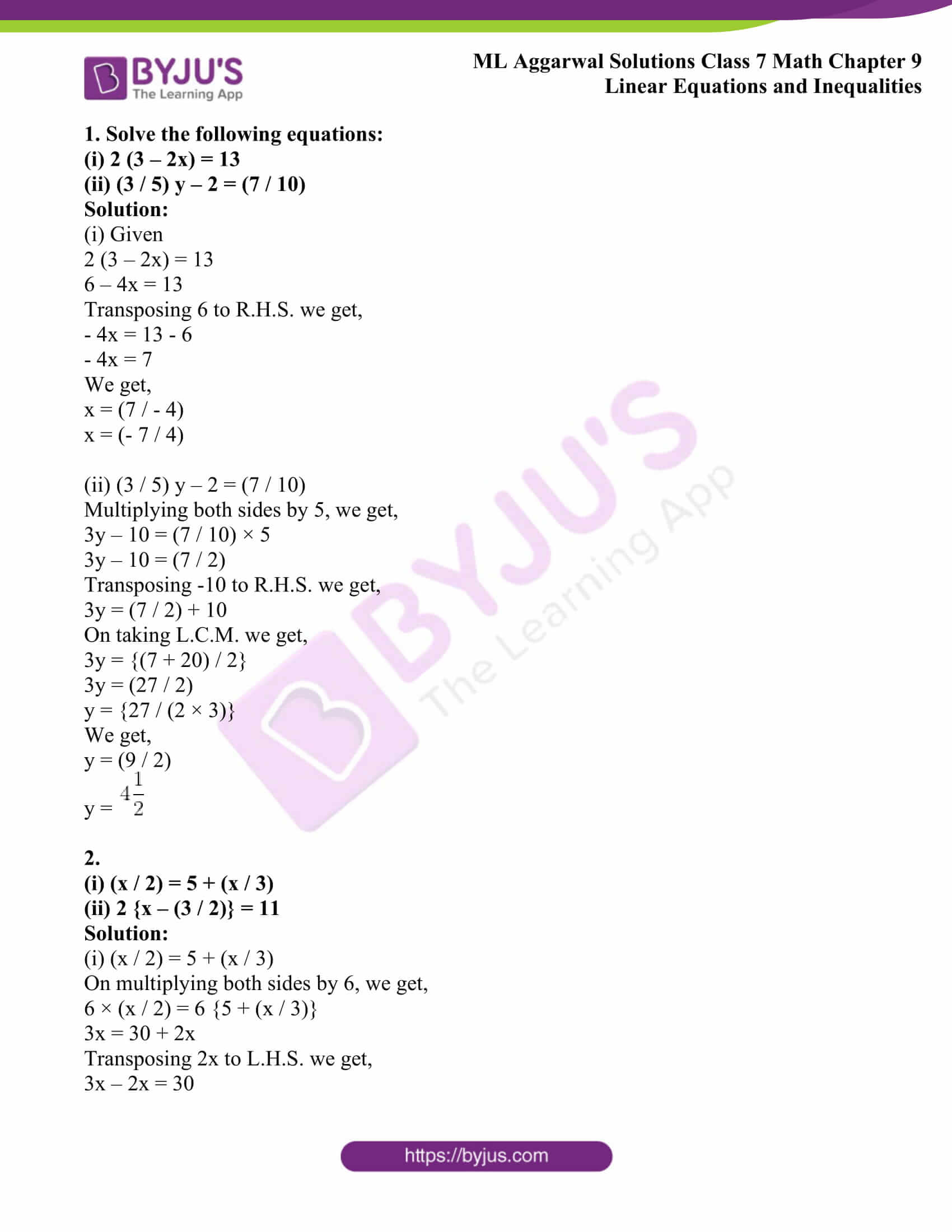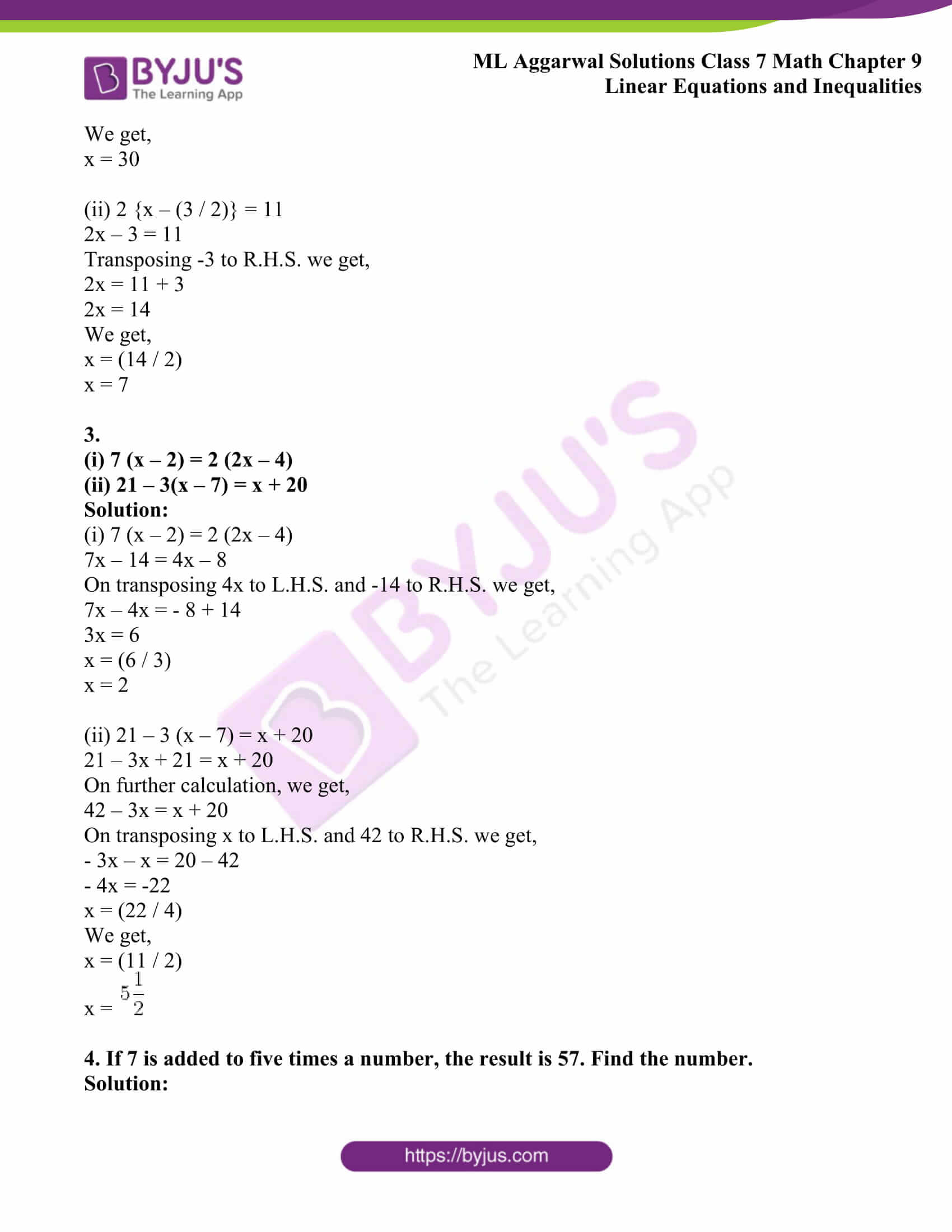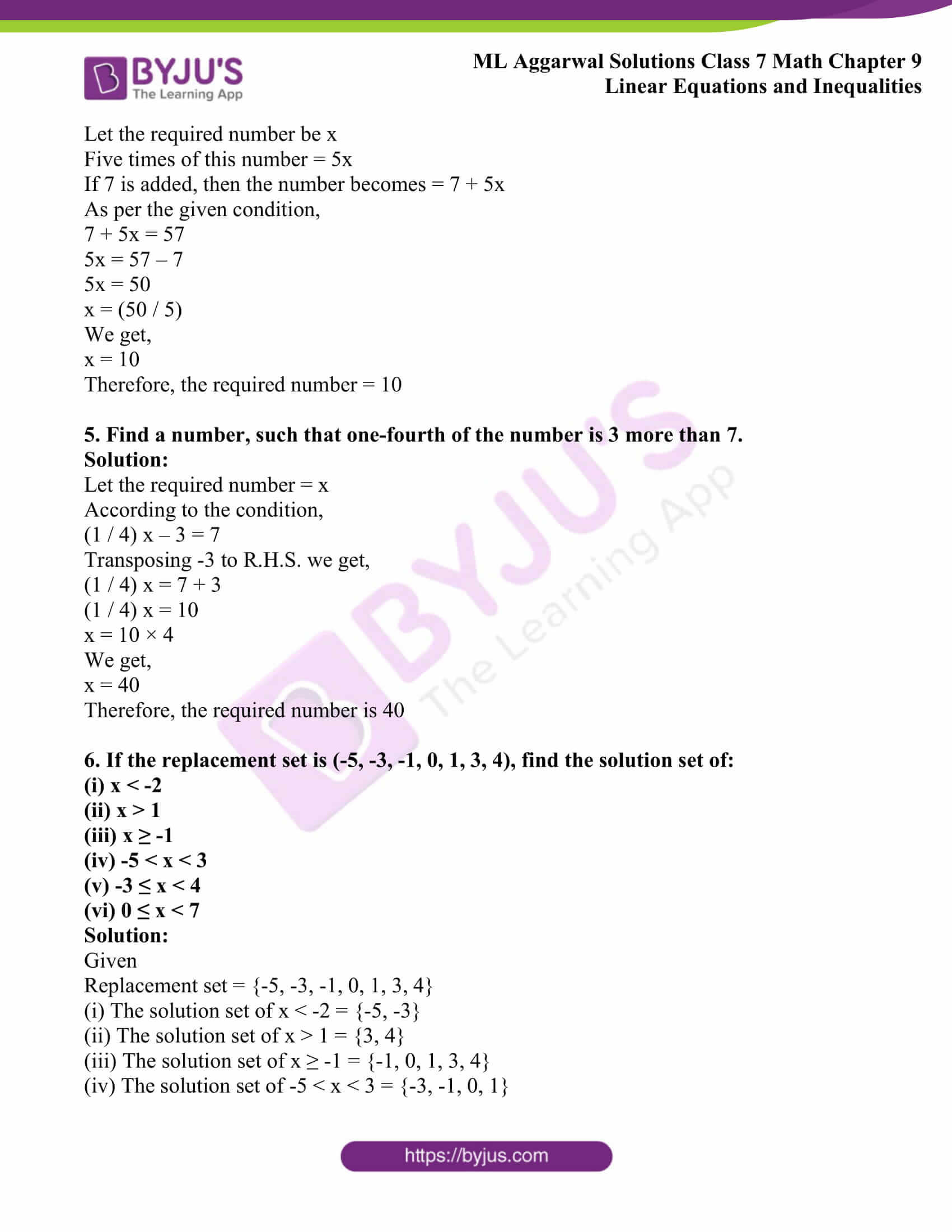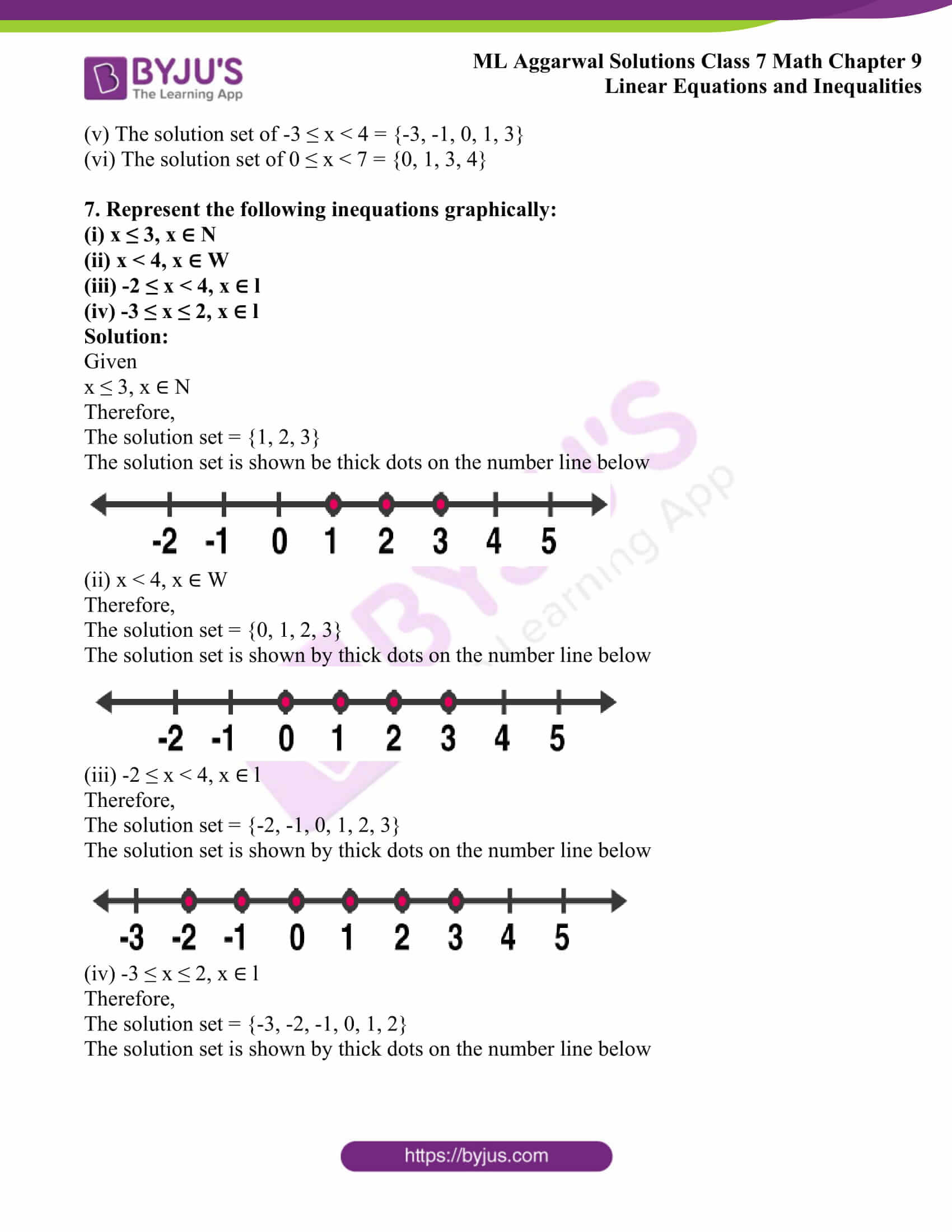## Access ML Aggarwal Solutions for Class 7 Maths Chapter 9 Linear Equations and Inequalities

1. Solve the following equations:

(i) 2 (3 – 2x) = 13

(ii) (3 / 5) y – 2 = (7 / 10)

Solution:

(i) Given

2 (3 – 2x) = 13

6 – 4x = 13

Transposing 6 to R.H.S. we get,

– 4x = 13 – 6

– 4x = 7

We get,

x = (7 / – 4)

x = (- 7 / 4)

(ii) (3 / 5) y – 2 = (7 / 10)

Multiplying both sides by 5, we get,

3y – 10 = (7 / 10) × 5

3y – 10 = (7 / 2)

Transposing -10 to R.H.S. we get,

3y = (7 / 2) + 10

On taking L.C.M. we get,

3y = {(7 + 20) / 2}

3y = (27 / 2)

y = {27 / (2 × 3)}

We get,

y = (9 / 2)

y =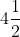2.

(i) (x / 2) = 5 + (x / 3)

(ii) 2 {x – (3 / 2)} = 11

Solution:

(i) (x / 2) = 5 + (x / 3)

On multiplying both sides by 6, we get,

6 × (x / 2) = 6 {5 + (x / 3)}

3x = 30 + 2x

Transposing 2x to L.H.S. we get,

3x – 2x = 30

We get,

x = 30

(ii) 2 {x – (3 / 2)} = 11

2x – 3 = 11

Transposing -3 to R.H.S. we get,

2x = 11 + 3

2x = 14

We get,

x = (14 / 2)

x = 7

3.

(i) 7 (x – 2) = 2 (2x – 4)

(ii) 21 – 3(x – 7) = x + 20

Solution:

(i) 7 (x – 2) = 2 (2x – 4)

7x – 14 = 4x – 8

On transposing 4x to L.H.S. and -14 to R.H.S. we get,

7x – 4x = – 8 + 14

3x = 6

x = (6 / 3)

x = 2

(ii) 21 – 3 (x – 7) = x + 20

21 – 3x + 21 = x + 20

On further calculation, we get,

42 – 3x = x + 20

On transposing x to L.H.S. and 42 to R.H.S. we get,

– 3x – x = 20 – 42

– 4x = -22

x = (22 / 4)

We get,

x = (11 / 2)

x =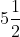4. If 7 is added to five times a number, the result is 57. Find the number.

Solution:

Let the required number be x

Five times of this number = 5x

If 7 is added, then the number becomes = 7 + 5x

As per the given condition,

7 + 5x = 57

5x = 57 – 7

5x = 50

x = (50 / 5)

We get,

x = 10

Therefore, the required number = 10

5. Find a number, such that one-fourth of the number is 3 more than 7.

Solution:

Let the required number = x

According to the condition,

(1 / 4) x – 3 = 7

Transposing -3 to R.H.S. we get,

(1 / 4) x = 7 + 3

(1 / 4) x = 10

x = 10 × 4

We get,

x = 40

Therefore, the required number is 40

6. If the replacement set is (-5, -3, -1, 0, 1, 3, 4), find the solution set of:

(i) x < -2

(ii) x > 1

(iii) x ≥ -1

(iv) -5 < x < 3

(v) -3 ≤ x < 4

(vi) 0 ≤ x < 7

Solution:

Given

Replacement set = {-5, -3, -1, 0, 1, 3, 4}

(i) The solution set of x < -2 = {-5, -3}

(ii) The solution set of x > 1 = {3, 4}

(iii) The solution set of x ≥ -1 = {-1, 0, 1, 3, 4}

(iv) The solution set of -5 < x < 3 = {-3, -1, 0, 1}

(v) The solution set of -3 ≤ x < 4 = {-3, -1, 0, 1, 3}

(vi) The solution set of 0 ≤ x < 7 = {0, 1, 3, 4}

7. Represent the following inequations graphically:

(i) x ≤ 3, x ∈ N

(ii) x < 4, x ∈ W

(iii) -2 ≤ x < 4, x ∈ l

(iv) -3 ≤ x ≤ 2, x ∈ l

Solution:

Given

x ≤ 3, x ∈ N

Therefore,

The solution set = {1, 2, 3}

The solution set is shown be thick dots on the number line below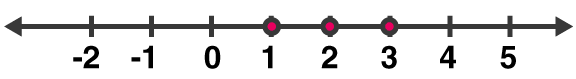(ii) x < 4, x ∈ W

Therefore,

The solution set = {0, 1, 2, 3}

The solution set is shown by thick dots on the number line below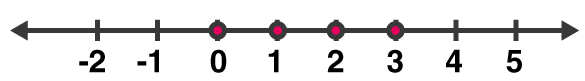(iii) -2 ≤ x < 4, x ∈ l

Therefore,

The solution set = {-2, -1, 0, 1, 2, 3}

The solution set is shown by thick dots on the number line below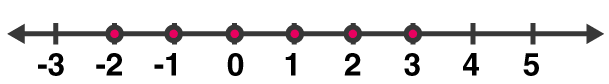(iv) -3 ≤ x ≤ 2, x ∈ l

Therefore,

The solution set = {-3, -2, -1, 0, 1, 2}

The solution set is shown by thick dots on the number line below